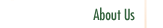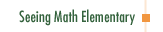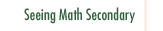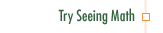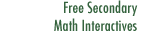Data Analysis and Probability:
Measures of Center

In Data Analysis and Probability: Measures of Center, we visit a classroom of students looking at ways of measuring and describing data and data sets. A note of interest—all the students in this class are English Language Learners (ELLs) or speakers of native languages other than English.

Participants in this course:

• Examine how students make sense of data "landmarks."
• Explore how initial knowledge informs and is changed by systematic observation.
• Explore how students learn to represent data using bar charts and line plots.
• Examine different questioning strategies to support concept clarification and discussion.
• Explore how nonverbal communication builds comfort and a sense of achievement in mathematics.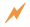Click to Get Seeing Math™ ElementaryCourse Listing Effective Questioning in the Mathematics Classroom Foundations of EffectiveMathematics Teaching Formative Assessment in the Mathematics Classroom Number & Operations: Division with Remainders Number & Operations: The Magnitude of Fractions Number & Operations: Broken Calculator Geometry: 2D and 3D Figures Geometry: Calculating Area of a Triangle Data Analysis and Probability: Using Data to Make Predictions Data Analysis and Probability: Measures of Center Pre-Algebra: Pan Balance Equations Pre-Algebra: Patterns and Functions